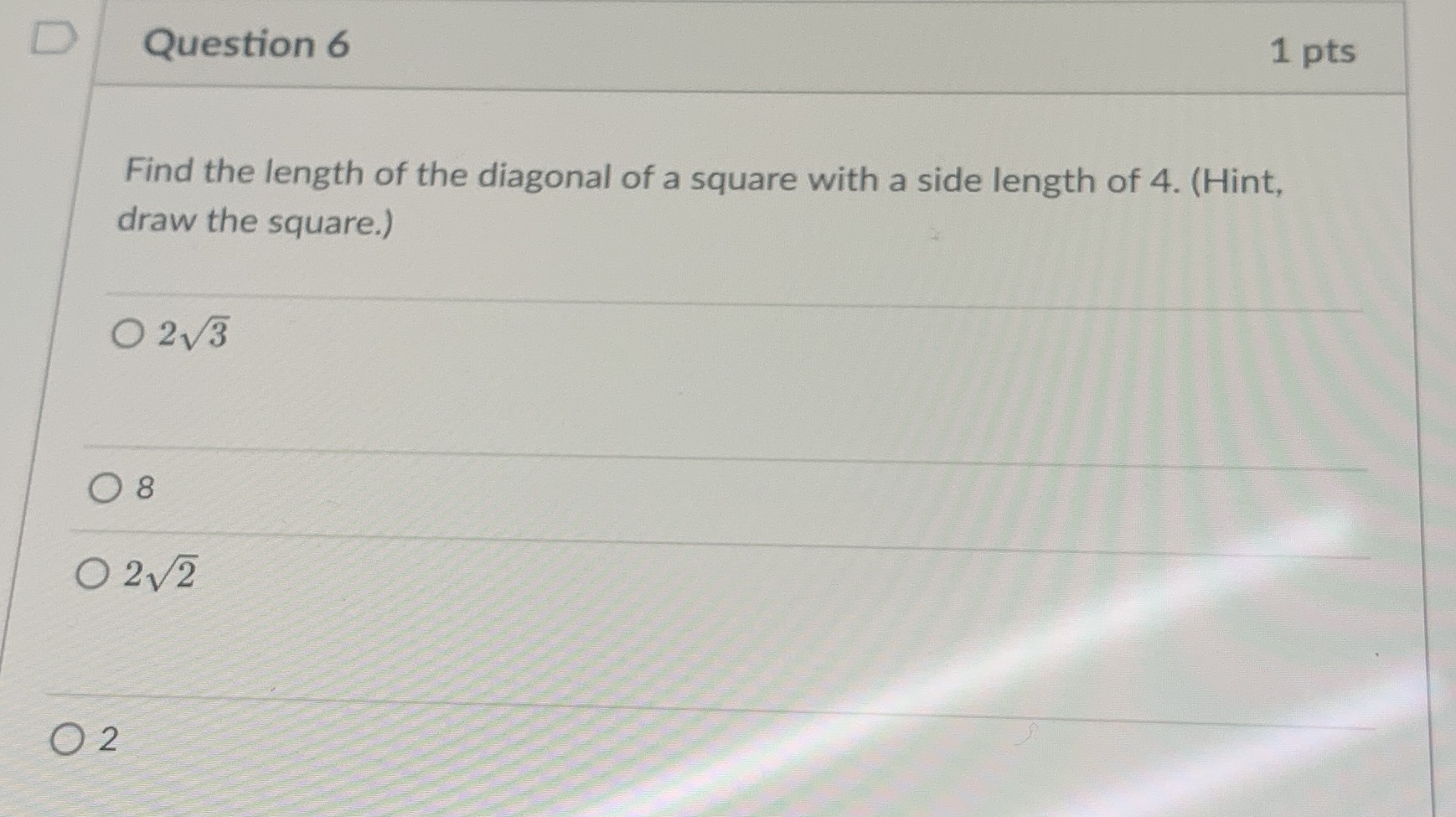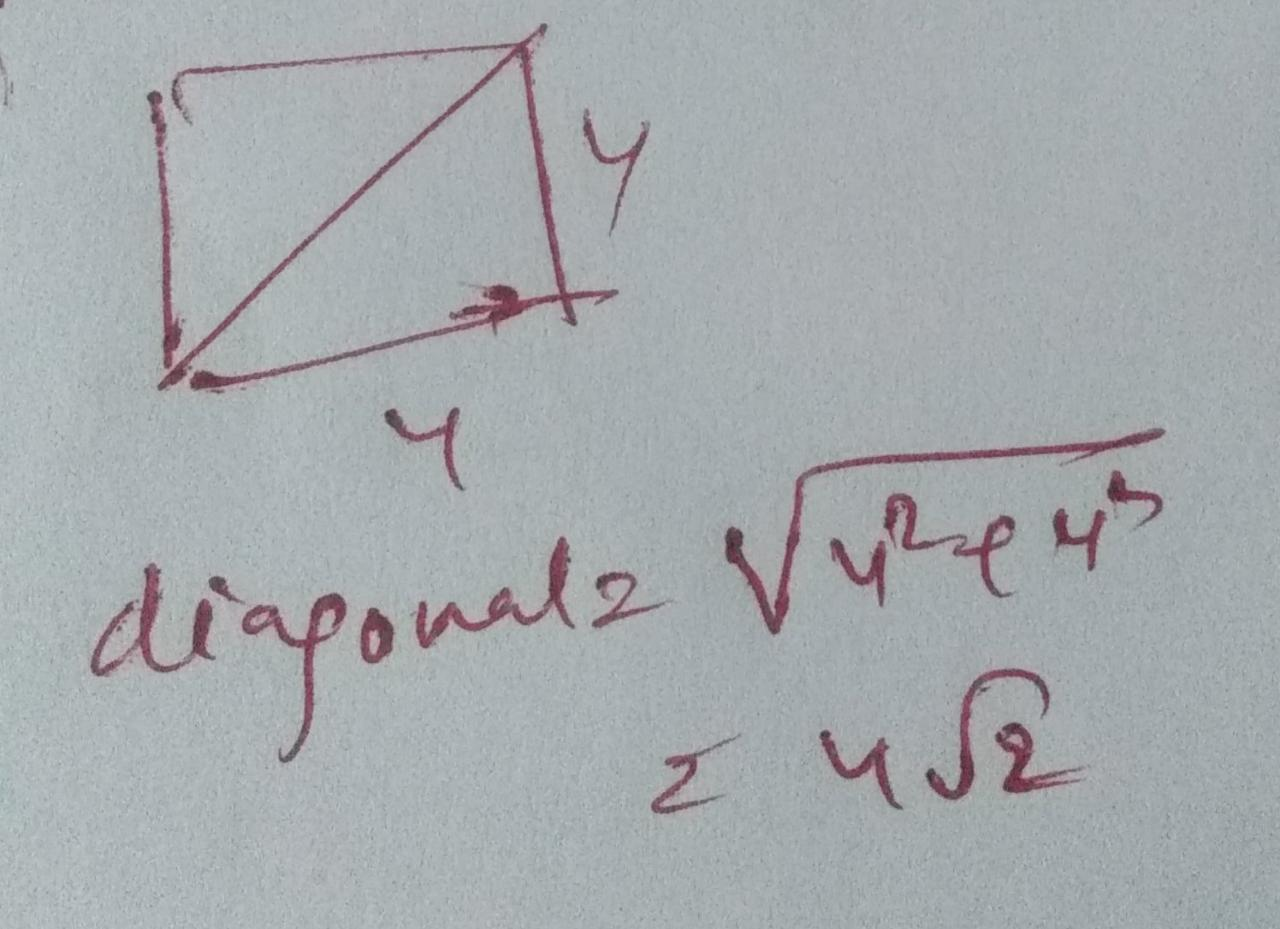### Still have math questions?

Trigonometry
QuestionQuestion $$6$$ Find the length of the diagonal of a square with a side length of $$4$$ . (Hint, draw the square.)

$$02 \sqrt { 3 }$$

$$08$$

$$12 \sqrt { 2 }$$

$$2$$